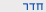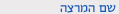שנה"ל תש"ףתורת המשחקים האלגוריתמית
Algorithmic Game Theory
0368-4483
מדעים מדויקים
קבוצה 01סמ'  ב'1400-1700001 רוזנברגשיעור ות פרופ פלדמן מיכל
ש"ס:  3.0

סילבוס מקוצר

Syllabus (tentative)

• Introduction: games, mechanism design, inefficiency of equilibrium and equilibrium computation
• Nash equilibrium and Nash’s theorem
• Zero-sum games: normal form and extensive form, minmax theorem, Yao’s principle
• Congestion games and potential games, pure NE existence and computation, best-response dynamics
• Inefficiency of equilibria: price of anarchy, price of stability, smoothness framework (extension to correlated equilibrium and regret minimization)
• Mechanism design basics: single-item auctions, Myerson’s lemma
• Algorithmic mechanism design: Multi-unit auctions: computation and communication
• VCG mechanisms
• Market models, equilibium, Welfare theorems, computational aspects

Course Requirements

• Class attendance is mandatory.
• Scribe notes (latex templatelatex tutorial)
• Problem sets: 2-3 problem sets will be given during the semeter. You should submit them in pairs.
• Final exam.
Course description
Algorithmic Game Theory

Instructor: Prof. Michal Feldman
Time & Place: Wednesday, 2-5pm (place TBA)
Prerequisites: probability theory, algorithms, complexity

Tentative list of topics:
Introduction to algorithms and games
Two-player zero-sum games (Minmax theorem)
Regret minimization and correlated equilibrium
Nash equilibrium
Mixed equilibrium and Nash theorem
Pure equilibrium
Equilibrium existence
Congestion games
Quality of equilibrium: price of anarchy and smoothness
Social choice and Mechanism Design
Introduction to social choice
Mechanism design without money (and approximation)
Social welfare maximization (VCG)
Combinatorial auctions
Revenue maximization (optimal mechanisms)
Markets and pricing
Walrasian equilibrium
First and second welfare theorems
Ascending price auctions

Final project (either a research project or a survey) – max 10 pages (possibly including presentation)
Scribe notes

Some references
Roughgarden, An algorithmic game theory primer (2008) http://theory.stanford.edu/~tim/papers/tcs08.pdf
Shoham, Communications of the ACM, Computer Science and Game Theory, http://robotics.stanford.edu/~shoham/www%20papers/CSGT-CACM080417.pdf
Halpern. Computer science and game theory: a brief survey
http://www.cs.cornell.edu/home/halpern/papers/csgt.pdfמדעים מדויקים
0368-4483-01 תורת המשחקים האלגוריתמית
Algorithmic Game Theory
שנה"ל תש"ף | סמ'  ב' | פרופ פלדמן מיכל

סילבוס מפורט/דף מידע
לצפייה בסילבוס נא ללחוץ כאן

להצהרת הנגישות